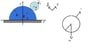# Hoop rolling on cylinder without slipping

• cacofolius

#### cacofolius

Hi everybody, I know this problem has been posted before, but it envolved Lagrangian methods which I haven't seen yet. I would appreciate any help.

1. Homework Statement

A small hoop is rolling without slipping on a bigger cylinder which is stationary. I need to write Newton's Laws and Torques, and find out at what angle the small cylinder falls off the bigger one.

##ζ=Iα##

##F=ma##

##I_{cm}= ma^2##

## The Attempt at a Solution

My restrain equation, since it rolls without slipping is that ##Φ=\frac{(R+a)}{a}θ## , because I'm taking θ=0 at the top.

##\hat{θ}) Fr- mg sinθ=m(R+a) \ddot{θ}##

##\hat{r}) N-mgcosθ=-m(R+a) \dot{θ}^2##

And around the center of mass of the small cylinder, the only force that makes a torque is friction, so

##ζ=I\ddot{Φ}##
##-aFr \hat{k} = -ma^2 \ddot{Φ} \hat{k} = -m a^2 \frac{(R+a)}{a} \ddotθ## So,

##Fr=m \frac{a^2}{a} \frac{(R+a)}{a} \ddotθ = m(R+a) \ddotθ##

And replacing this in my theeta equation I get:

##\hat{θ}) m(R+a) \ddot{θ} - mg sinθ=m(R+a) \ddot{θ}##

And this is where I get confused, becuse I get ## mgsinθ=0## I now I'm making a silly mistake somewhere, like the direction of a vector or something, but I cannot see it. Thanks in advance.

#### Attachments

•cylinder.jpg
14.8 KB · Views: 856

For starters, I suggest that you reverse the direction of the theta unit vector, so that it points down slope rather than up slope. The system remains right-handed, but this will make things work out easier.

Now, what do you mean by the angle phi? Is it the absolute rotation, or the rotating of the contact point on the small cylinder?

Also, it looks like you have said that the radial force is the cause of the torque driving the angular acceleration of the small cylinder. This is wrong.

##\hat{θ}) 2m(R+a) \ddot{θ} + mg sinθ=0 ##

which is nice for small angle approximation and describing the oscillatory movement.
And Fr meant friction force. Sorry it took long for me to answer back. Thanks for your help!

What is theta-hat?

My restrain equation, since it rolls without slipping is that ##Φ=\frac{(R+a)}{a}θ## , because I'm taking θ=0 at the top.
How are you defining Φ? I assume it's the rotation of the hoop about its own centre, and in the diagram the black arrow represents a radius to the point that was originally at the bottom, i.e. arrow pointing down, yes?
The easiest way with these problems is generally to start with conservation of energy, if appropriate. That will give you the velocity as a function of theta.
## \hat {\theta} \right) 2m(R+a) \ddot {\theta} + mg \sin \theta = 0##
Better, but still seems to have a sign wrong. Wouldn't that make ##\ddot{θ}## negative?
small angle approximation
You don't need such.
describing the oscillatory movement
What oscillation?
What is theta-hat?
It's indicating that the equation relates to tangential direction.